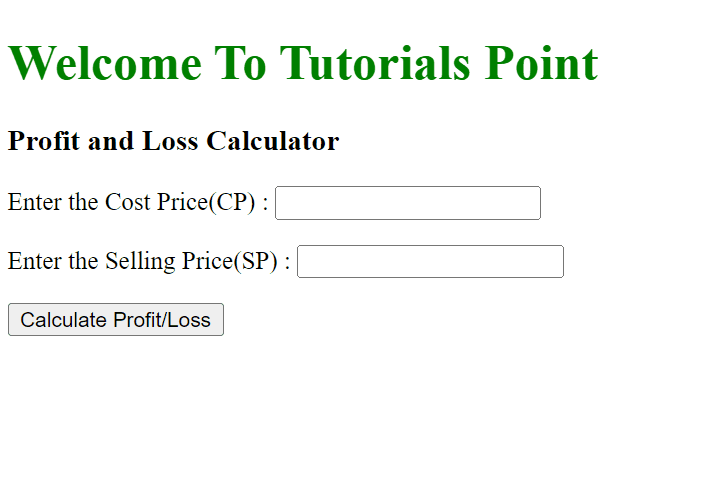# How to Create a Profit and Loss Calculator using HTML, CSS, and JavaScript?

In this article, we are going to create a calculator using JavaScript We will be using the basic math formulas to calculate the profit and loss. We will be returning the result in percentage along with the actual values.

To calculate the Profit and Loss we need two things that are: CP (Cost Price) and SP (Selling Price).

Formulas to Calculate Profit and Loss −

• Profit: SP – CP

• Profit Percentage: Profit/CP * 100

• Loss: SP – CP

• Loss Percentage: Loss/CP * 100

## Example 1

In the example below, we have created a calculator that will calculate the profit and loss percentage as per the cost price and the selling price.

On clicking the Calculate button, it will call the function that will get the CP & SP inputs and then calculate the profit and loss.

#Filename: index.html

<!DOCTYPE html>
<html lang="en">
<title>Profit and Loss Calculator</title>
<body>
<div class="plcalculate">
<h1 style="color: green;">
Welcome To Tutorials Point
</h1>
<h3>Profit and Loss Calculator</h3>
<p>Enter the Cost Price(CP) :
<input class="cp" type="number" />
</p>
<p>Enter the Selling Price(SP) :
<input class="sp" type="number" />
</p>
<button onclick="Calculate()">Calculate Profit/Loss</button>
<p>
<h2 class="loss"></h2>
<h2 class="lossPercentage"></h2>
<h2 class="nothing"></h2>
</p>
</div>
<script>
function Calculate(){
const CP= document.querySelector(".cp").value;
const SP= document.querySelector(".sp").value;
const profitLoss=document.querySelector(".loss");
const percentage=document.querySelector(".lossPercentage");
const nothing=document.querySelector(".nothing");
profitLoss.innerHTML="";
percentage.innerHTML="";
nothing.innerHTML="";
const diff=SP - CP;
if(diff>0){
const profit_percent= ((diff/CP)*100).toFixed(2);
profitLoss.innerHTML="It is a Profit of: INR "+ diff;
percentage.innerHTML="Total Profit Percentage : "+
profit_percent;
} else if(diff<0){
const loss_percent= ((Math.abs(diff)/CP)*100).toFixed(2);
profitLoss.innerHTML="It is a Loss of: INR "+ Math.abs(diff);
percentage.innerHTML="Total Loss Percentage : "+ loss_percent;
} else if(diff==0){
nothing.innerHTML="No Profit No Loss";
}
}
</script>
</body>
</html>

## Output

On successful execution of the above program, it will produce a Profit and Loss calculator. You can enter Cost Price and Selling Price. After entering the Cost Price and Selling Price, you can calculate the profit or loss by clicking the button “Calculate Profit/Loss”.